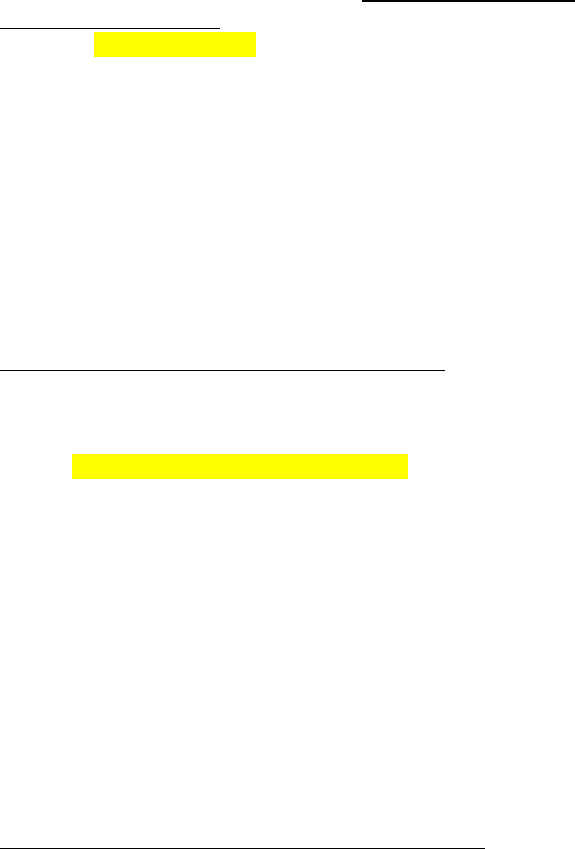Textbook Notes (270,000)
CA (160,000)
U of G (10,000)
SOAN (400)
Chapter 5

SOAN 3120 Chapter Notes - Chapter 5: Standard Deviation, Dependent And Independent Variables, Scatter Plot

Department
Sociology and Anthropology
Course Code
SOAN 3120
Professor
Andrew Hathaway
Chapter
5

This preview shows half of the first page. to view the full 3 pages of the document.Chapter 5:
Regression Lines:
A regression line is a straight line that describes how a response variable y
changes as an explanatory variable x changes. We often use a regression line
to predict the value of y for a given value of x
When y is the response variable (on the vertical axis) and x is an explanatory
variable (plotted on the horizontal axis) a straight line relating y to x has an
equation of the form:
oY = a + bx
oB = the slope (the amount by which y changes when x increases by
one unit)
oA = the intercept ( the value of y when x = 0)
The size of the slope depends on the units in which we measure the 2
variables
The Least-Squares Regression Line:
In most cases no line will pass through all points on a scatterplot
A good regression line makes the vertical distance of the points from the line
as small as possible
Least-Squares Regression Line: of y on x is the line that makes the sum of the
squares of the vertical distances of the data points from the line as small as
possible
Equation:
oWe have data on an E variable (x) and a R variable (y) for n individuals
oFrom the data calculate the means for x and y and the standard
deviations and their correlation (r)
oWith slope: y(hat) = a + bx
oB = r (sy/sx)
oAnd intercept: a = mean of y – b(mean of x)
We write y-hat in the equation of the regression line to emphasize that the
line gives a predicted response y-hat to any x
The predicted response will usually not be exactly the same as the actually
observed response to y
Least squares regression line have man convenient properties, and some
facts:
1. The distinction between E and R variables is essential in regression
a. It makes the distance of the data point from the line small in the y
direction
b. If we reverse the roles of the 2 variables we get a di3erent least
squares regression
2. There is a close connection between correlation and the slope of the least
squares line
a. The slop and the correlation always have the same sign , if a
scatterplot shows a positive association then both b and r are
positive
b. A change in one standard deviation in x corresponds to a change of
r standard deviations in y